# Complex floor and a surprising pattern

The floor of a real number x, written ⌊x⌋, is the largest integer less than or equal to x. So, for example, ⌊π⌋ = 3 and ⌊-π⌋ = -4.

The previous post applied the floor function to a complex number z. What does that mean? You can’t just say it’s the largest integer  less than z because the complex numbers aren’t ordered; there’s no natural way to say whether one complex number is less than or greater than another.

The post was using Mathematica code, and Mathematica defines the floor of a complex number by applying the function to the real and imaginary parts separately. That is, if x and y are real numbers, then

x + iy⌋ = ⌊x⌋ + iy⌋.

That lets you take the floor of a complex number, but is it useful? I wondered how many identities that hold for floors of real numbers extend to complex numbers when the floor is defined as above, so I looked at the chapter in Concrete Mathematics that has a bunch of identities involving floors and ceilings to see which ones extend to complex numbers.

Any identity where the real an imaginary parts don’t interact should extend trivially. For example, for real x and integer n,

x + n⌋ = ⌊x⌋ + n.

This extends to the case where x is complex and n is a (complex) integer . Addition works on real and imaginary components separately, just like Mathematica’s definition of floor.

But here’s one that’s much more interesting. For positive x,

⌊√⌊x⌋ ⌋ = ⌊√x⌋.

Taking square roots deeply intertwines the real and imaginary parts of a number . Does the identity above hold for complex inputs?

I wrote some Python code to test this, and to my great surprise, the identity often holds. In my first experiment, it held something like 84% of the time for random inputs. I expected it would either rarely hold, or hold say half the time (e.g. in a half-plane).

My first thought was to plot 1,000 random points, green dots where the identity holds and red stars where it does not. This produced the following image.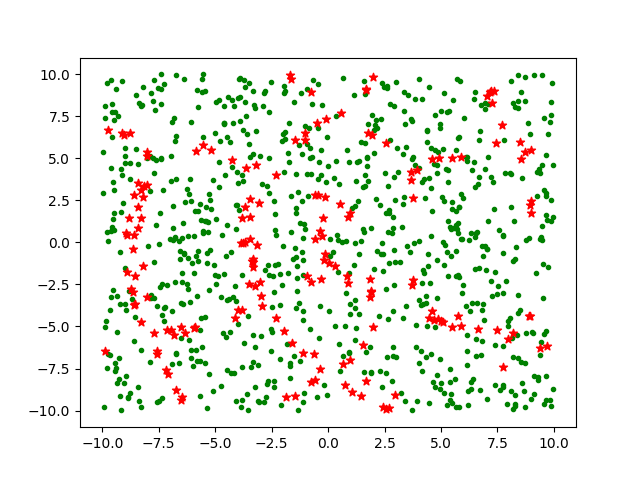About 16% of the points are red and the rest green. In this plot there’s no apparent pattern to the red points.

Since I’m sampling uniformly throughout the square, there’s no reason to plot both where the identity holds and where it doesn’t. So for my next plot I just plotted where the identity fails.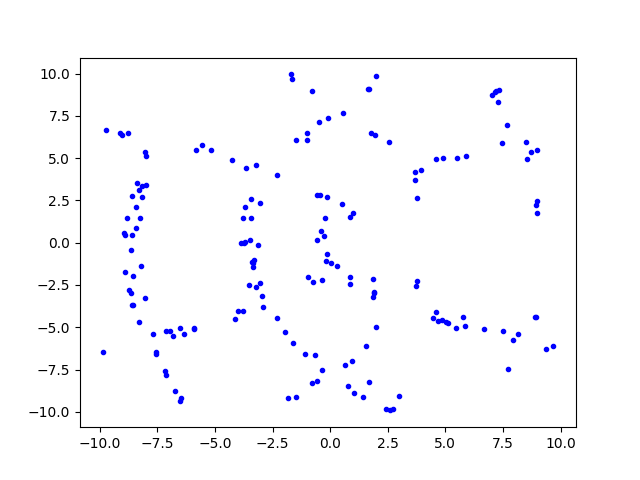That’s a little  clearer. To make it clearer, I rerun the plot with 10,000 samples. (That’s the total number of random samples tested, about 16% of which are plotted.)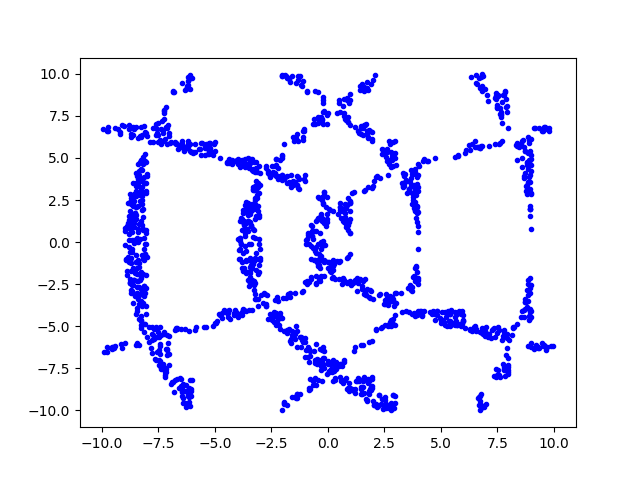Then to improve the resolution even more, I increased the number of samples to 1,000,000.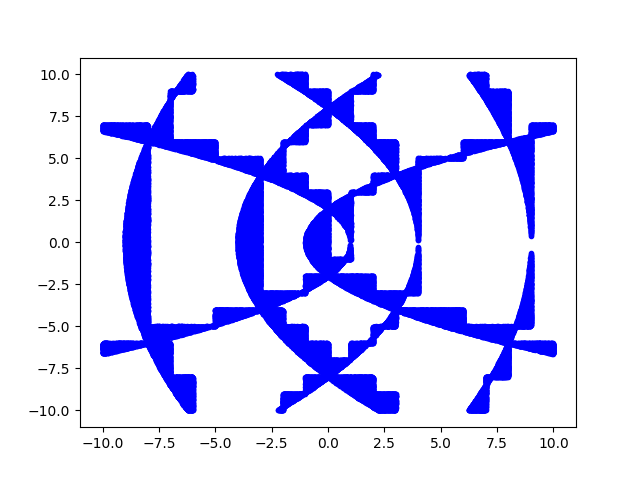It might not be too hard to work out analytically the regions where the identity holds and doesn’t hold. The blue regions above look sorta like parabolas, which makes sense because square roots are involved. And these parabola-like curves has ziggurat-like embellishments, which makes sense because floors are involved.

## Update

Define

f(z) = ⌊√⌊z⌋ ⌋ – ⌊√z⌋.

The graphs above plotted the set of zs for which f(z) ≠ 0. We can see more information by plotting f itself. The function only takes on five possible values: 0, 1, i, -1, and –i. I plotted these as white, orange, green, blue, and red.## Related posts

 You could extend the meaning of “integer” to a complex number with integer real and imaginary parts, commonly known as a Gaussian integer.

 I suspect Mathematica’s definition is not common. I don’t know of any other source that even defines the floor of a complex number, much less defines it as Mathematica does.

 You may be wondering what’s going on with the square root. Complex numbers have two square roots, so which one(s) are we using? I’m doing what Python does, taking the principle branch. That is, using the analytic continuation of square root that extends from the real axis to the complex plane with the negative real axis excluded.

# Plotting the Gauss map

A recent post looked at an example from one of Michael Trott’s tomes. This post looks at another example from the same tome.

Trott made a contour plot of the Gauss mapover the complex plane. I copied his code (almost) and reproduced his plot.

    ContourPlot[
Abs[1/(x + I y) - Floor[1/(x + I y)]],
{x, -1.1, 1.1}, {y, -1.1, 1.1},
PlotPoints -> 40, ColorFunction -> Hue,
ContourStyle -> {Thickness[0.00001]}
]


This produced the following image.The original code set PlotPoints to 400, and it was taking forever. I got impatient and set the parameter to 40. Looking at the image in the book, it seems the plot with the parameter set to 400 is very similar, except there’s a black tangle in the middle rather than a white space.

In 2004 when Trott wrote his book, Mathematica did not have a command for plotting functions of a complex variable directly, and so Trott rolled his own as a function of two real variables.

Here’s a plot of the same function using the relatively new ComplexPlot function.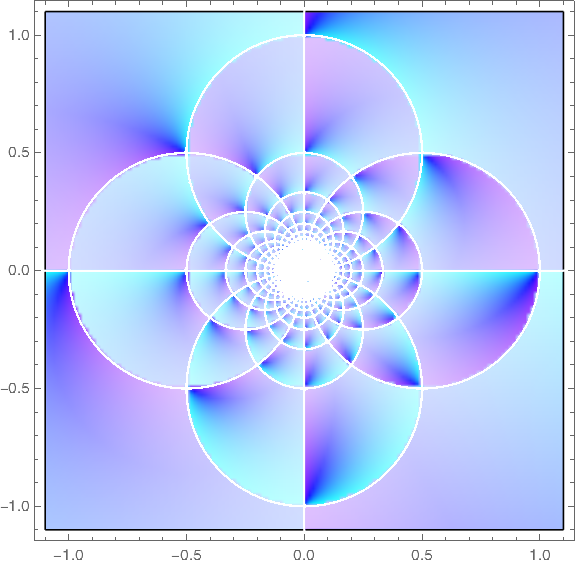Here’s the code that produced the plot.

    ComplexPlot[
(1/z - Floor[1/z]),
{z, -1.1 - 1.1 I, 1.1 + 1.1 I},
ColorFunction -> Hue, PlotPoints -> 400
]


## Update

What does it mean to apply the floor function to a complex number? See the next post.

The plots above were based on Mathematica’s definition of complex floor. Another definition gives different plots.

    aplfloor[z_] :=
With[ {x = Re[z] - Floor[Re[z]], y = Im[z] - Floor[Im[z]], g = Floor[z]},
If[x + y > 1, If[x >= y, g + 1, g + I], g]]


This leads to different plots of the Gauss map.

Vintage: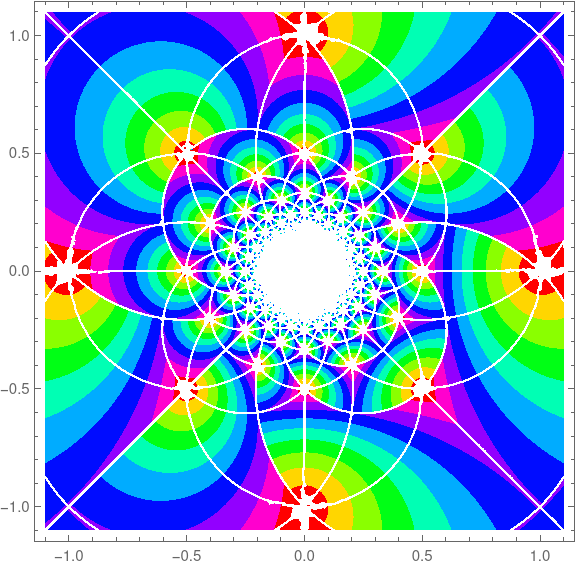And new style: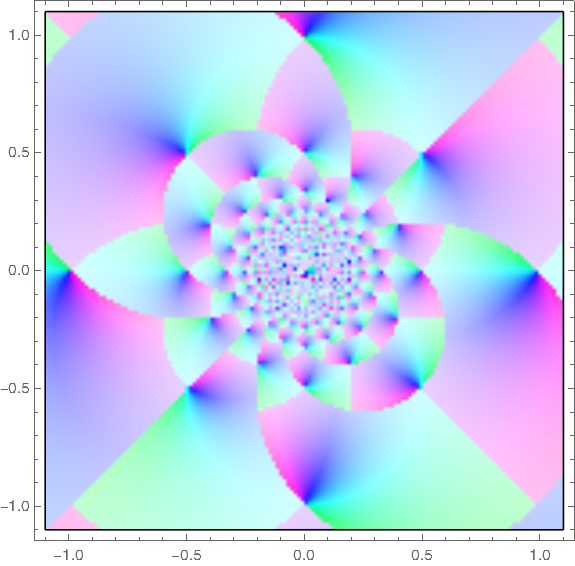# Solving for Möbius transformation coefficients

Möbius transformations are functions of the formA Möbius transformation is uniquely determined by its values at three points. Last year I wrote a post that mentioned how to determine the coefficients of a Möbius transformation. There I said

The unique bilinear transform sending z1, z2, and z3 to w1, w2, and w3 is given byPlug in your constants and solve for w = f(z).

This is correct, but it still leaves a bit of work to do. In a particular case it’s not hard to find the coefficients, but it would be harder to find the coefficients in general.

There is an explicit formula for each of the parameters a, b, c, and d given the specified points z1, z2, z3 and their images w1, w2, w3 which we will present shortly. We could easily code-up these formulas except for one complication: we may want one of our inputs our outputs to be ∞. This is not merely an edge case: in applications, say to signal processing, you often want to specify the location of poles.

# Constructing bilinear transformations

The previous post was a visual introduction to bilinear transformations, a.k.a. Möbius transformations or fractional linear transformations. This post is a short follow-up focused more on calculation.

A bilinear transformation f has the form## Inverse

The inverse of f is given byThe transformation f is defined everywhere except at z = –d/c, and its inverse is defined everywhere except at w = a/c.

So f takes the complex plane minus one point to the complex plane minus one point. Or an elegant way of thinking about it is to think of f and g as functions on a sphere by adding a point at infinity. Then we say## Determining by three points

Bilinear transformations have three degrees of freedom. That is, you can pick three values in the domain and specify three places for them to go in the range. The unique bilinear transform sending z1, z2, and z3 to w1, w2, and w3 is given byPlug in your constants and solve for w = f(z).

Update: Here are explicit formulas for the coefficients.

## Example

For example, let’s look at the smiley face example from the previous post.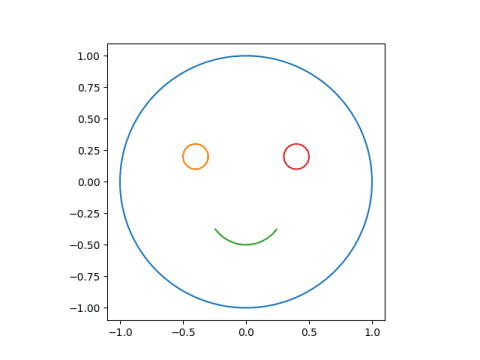We’ll pick three points on the face and three places for them to go.

Let’s say we want the center of the face to stay put, mapping 0 to 0. Next let’s pick two places for the center of the eyes to go. These are at
±0.4+.2ji. Say we want the left eye to go down a little to -0.4 and the right eye to go up and over a little to 0.5 + 0.3i.

I used Mathematica to solve for the parameters.

    {z1, z2, z3} = {0, -2/5 + I/5, 2/5 + I/5}
{w1, w2, w3} = {0, -2/5, 1/2 + 3 I/10}
Solve[(w - w2) (w3 - w1)/((w - w1) (w3 - w2)) ==
(z - z2) (z3 - z1)/((z - z1) (z3 - z2)), w]


This says the parameters are, in Python notation,

    a = -72 - 16j
b = 0
c = 30 - 35j
d = -75


Using the code from the previous post we can verify that this transformation does what we designed it to do.

    print(mobius(0, a, b, c, d))
print(mobius(-0.4 + 0.2j, a, b, c, d))
print(mobius(0.4 + 0.2j, a, b, c, d))


and we can take a look at the result.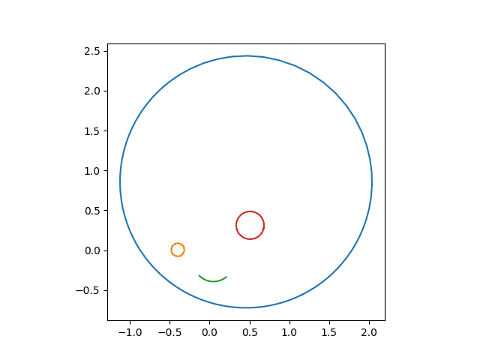# Circles to Circles

This post expands on something I said in passing yesterday. I said in the body of the post that

… the image of a circle in the complex plane under a Möbius transformation is another circle.

and added in a footnote that

For this to always be true, you have to include a line as a special case of a circle, a circle of infinite radius if you like.

This post will illustrate these statements with Python code and plots. First, some code for drawing circles and other curves in the complex plane.

    from numpy import exp, pi, linspace
import matplotlib.pyplot as plt

θ = linspace(0, 2*pi, 200)

def plot_curves(curves):
for c in curves:
plt.plot(c.real, c.imag)
plt.axes().set_aspect(1)
plt.show()
plt.close()


Next, code for Möbius transformations, and the particular Möbius transformation we’ll use in our plots.

    def mobius(z, a, b, c, d):
return (a*z + b)/(c*z + d)

def m(curve):
return mobius(curve, 1, 2, 3, 4)


Now we’ll plot three circles and their images under the Möbius transformation

m(z) = (z + 2)/(3z + 4)

with the following code.

    circles = [circle(1, 0), circle(2, 0), circle(2, 2)]
plot_curves(circles)
plot_curves([m(c) for c in circles])


This producesand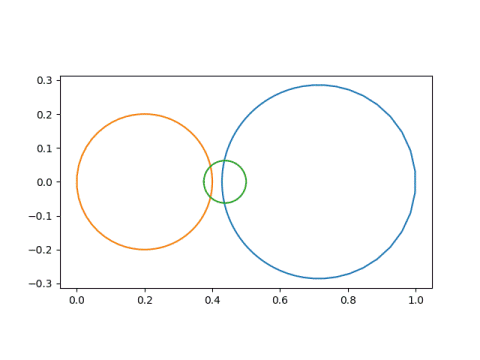Notice that the first circle, in blue, started out as the smallest circle and was contained inside the second circle, in orange. But in the image, the blue circle became the largest, and is no longer inside the orange circle. That is because our Möbius transformation has a singularity at -4/3, and things get turned inside-out around that point.

Next we’ll look at an example of lines being mapped to lines.

    line = linspace(-100, 100, 600)
curves = [line, 1j*line - 4/3]
plot_curves(curves)
plot_curves([m(c) for c in curves])


This produces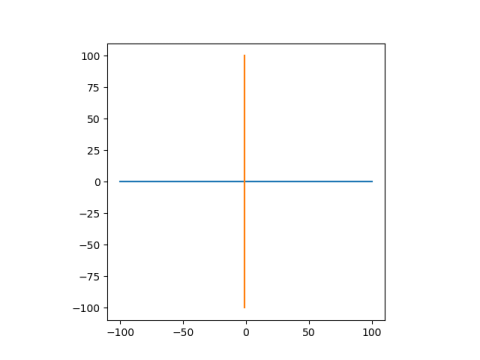and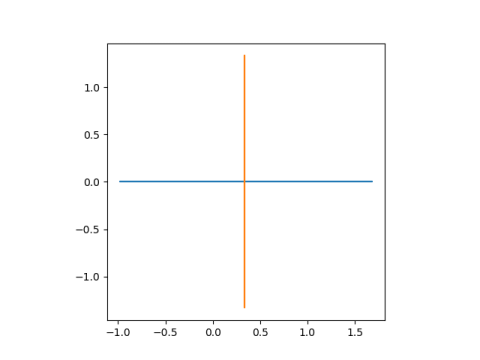These lines are mapped to lines because they both pass through the singularity at -4/3. The real axis, in blue, is mapped to itself. The line -4/3 + iy, is shifted over to have real part 1/3.

Finally, lets look at lines being mapped to circles. Since the inverse of a Möbius transformation is another Möbius transformation, this example also shows that circles can be mapped to lines.


lines = [1j*line - 4, 1j*line + 4, line - 4j, line + 4j]
plot_curves(lines)
plot_curves([m(c) for c in lines])


This produces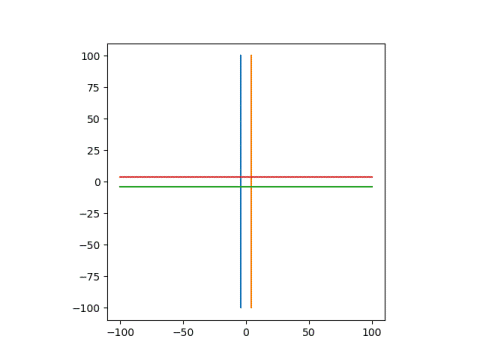and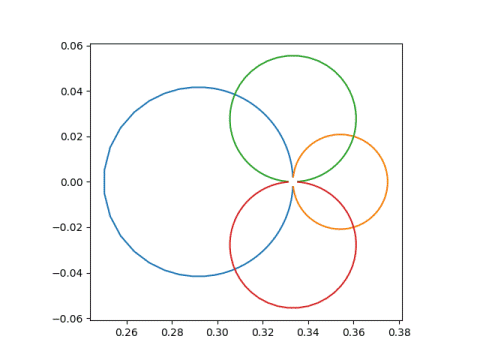Note that the circles don’t quite close. That’s because my line only runs from -100 to 100, not -∞ to ∞. The gap in the circles is at 1/3, because that’s the limit of our transformation (z + 2)/(3z + 4) as z goes to ±∞.

## Smiley faces

To illustrate things further, I’d like to look at a smiley face and what happens to it under different Möbius transformations.Here’s the code to draw the original face.

    dash = linspace(0.60, 0.90, 20)
smile = 0.3*exp(1j*2*pi*dash) - 0.2j
left_eye  = circle(0.1, -0.4+.2j)
right_eye = circle(0.1,  0.4+.2j)
face = [circle(1, 0), left_eye, smile, right_eye]


Next, let’s subject this face to the Möbius transformation with parameters (1, 0, 1, 3). The singularity is at -3, outside the face and fairly far away.Next we’ll use parameters (1, 0, 1, -1+1j), which has a sigularity at 1 – i, closer to the face, and hence more distortion.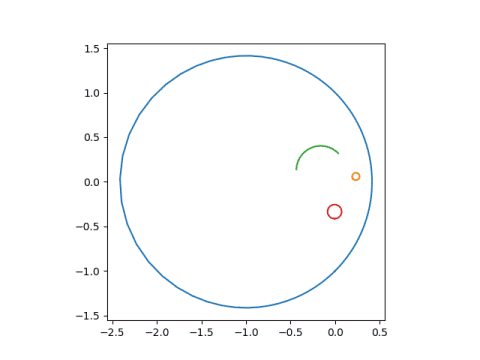Now we use parameters (1, 0, 3, 1), putting the singularity at -1/3, inside the face.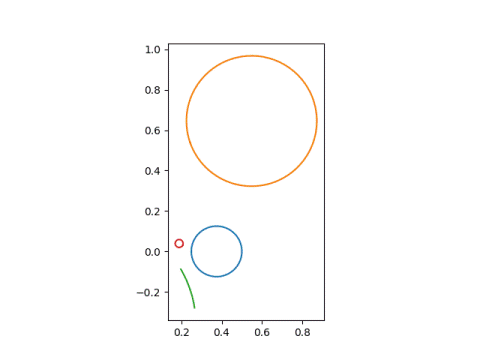Finally, we look at parameters (1, 0, 1, 0.4-0.2j), putting the singularity inside the left eye.The next post explains how to pick the parameters of a Möbius transformation to make points go where you want.

# Schwarzian derivative

There are many ways the basic derivative can be generalized: partial derivatives, directional derivatives, covariant derivatives, etc. These all reduce to the basic derivative under the right circumstances.

The Schwarzian derivative is not like that. It’s not a generalization of the familiar derivative but rather a differential operator analogous to a derivative. The Schwarzian derivative of a function f is defined  asTo understand the motivation behind such an arbitrary-looking definition, we need to first look at functions of the formcalled Möbius transformations, or more descriptively, fractional linear transformations . These transformations are very important in complex analysis and have a lot of interesting properties. For example, the image of a circle in the complex plane under a Möbius transformation is another circle .

Möbius transformations are to the Schwarzian derivative roughly what constants are to the ordinary derivative. A function is a Möbius transformation if and only if its Schwarzian derivative is zero.

Since the Schwarzian derivative is defined in terms of ordinary derivatives, adding a constant to a function doesn’t change its Schwarzian derivative. Furthermore, the Schwarzian derivative is defined in terms of the ratio of ordinary derivatives, so multiplying a function by a constant doesn’t change its Schwarzian derivative either.

Even more generally, applying a Möbius transformation to a function doesn’t change its Schwarzian derivative. That is, for a fractional linear transformation like g(z) abovefor any function f. So you can pull Möbius transformations out of a Schwarzian derivative sorta like the way you can pull constants out of an ordinary derivative. The difference though is that instead of the Möbius transformation moving to the outside, it simply disappears.

You can think of the Schwarzian derivative as measuring how well a function can be approximated by a Möbius transformation. Schwarzian derivatives come up frequently in applications of complex analysis, such as conformal mapping.

## More kinds of derivatives

 The Schwarzian derivative of a constant function is defined to be zero.

 Möbius transformations require adbc to not equal zero.

 For this to always be true, you have to include a line as a special case of a circle, a circle of infinite radius if you like. If you don’t like that definition, then you can rephrase the statement above as saying Möbius transformations map circles and lines to circles and lines.

# Not even closeVery often what cannot be done exactly can be done approximately. For example, most integrals cannot be computed in closed form, but they can be calculated numerically as closely as you’d like. But sometimes things are impossible, and you can’t even come close.

## An impossible assignment

When I was in college, I had a friend who had a job in an engineering lab. The director of his lab had asked him to find an analytic analog to a smoothed indicator function. (I’ll say in more detail what that means shortly.) He mentioned the problem to me, and I told him there’s a theorem that says his assignment is impossible .

My friend was not deterred by my answer, confident that as an engineer he could find a practical way to do what mathematicians say can’t be done. That’s often a useful attitude, though not in this instance. Looking back, I can see how I could have given him a more useful reply than simply saying his task couldn’t be done; I could have explained why it cannot even be done approximately.

## No exact plateaus

The indicator function of an interval is a function that takes on the value 1 on the interval and 0 everywhere else. This is a discontinuous function, but it can be approximated by a smooth function. Given a little transition zone on either end, you can have the function be zero on one side, one on the other, and ramp up smoothly in between. You can do this with infinitely differentiable functions, so why can’t you do something similar with analytic functions?

An analytic function can be expressed as a power series, and a power series is determined by its values in a small neighborhood of where it is centered. If you look at a patch where the function is zero, all its derivatives are zero, and so all the series coefficients are zero, and the function is zero. So the function’s value is zero everywhere. An analytic function can’t have a plateau without being flat everywhere.

## No approximate plateaus

But can an analytic function have an approximate plateau? Can you construct an analytic function that is nearly 1 on some region, say a disk, and nearly 0 outside of some thin boundary around the disk ? In more picturesque language, can you construct an analytic function whose absolute value looks like Devil’s Tower in the photo above?

The barrier to creating something like Devil’s Tower is the maximum modulus principle. It says that the absolute value of an analytic function cannot have an interior maximum; the maximum always occurs on the boundary.

Suppose you’re trying to construct a function f(z) such that |f(z)| is a approximately zero within a radius 1 of the origin and approximately 0 outside a disk of radius 2. The first part is possible but the second part is not. The function f(z) cannot be perfectly flat on top without being constant everywhere (Liouville’s theorem) and the maximum of |f(z)| over the unit disk must occur somewhere on the rim of the disk (maximum modulus principle). However, it could be that f doesn’t vary much on the disk, and so there’s not much difference between its maximum and minimum over the disk.

Now consider the disk of radius 2. Somewhere on the rim, the circle of radius 2, |f(z)| must be larger than it was on the unit disk, or else |f(z)| would have an interior maximum, which the maximum modulus principle says cannot happen. |f(z)| might be small along parts of the circle of radius 2, but somewhere on that circle it is approximate 1 or larger.

## Fake plateaus

Sometimes you will see plots of analytic functions that do look flat on top, but that’s because singularities have been chopped off. Here’s such a plot from a blog post in a while back, a plot of Weierstrass’ elliptic function. The white plateaus are artifacts of cutting infinite values to fit in a finite box.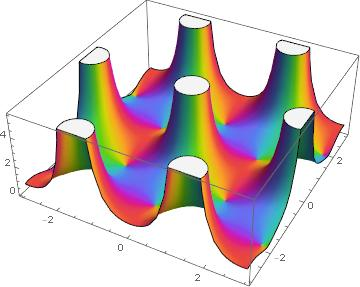## Related posts

 I wondered for a second how my friend’s supervisor couldn’t know this, then I realized it was probably a setup. His supervisor had given him an impossible task so that he’d struggle with it and learn why it was impossible.

 You could construct an analytic function that approximates a plateau along a one-dimensional slice, say along the real axis, but that approximation cannot be good in all directions.

# Plotting complex functions

I wrote a blog post of sorts, spread over several tweets, about plotting functions of a complex variable.

Here are a couple of the images from the tweet stream. First Weierstrass’s elliptic function.And a phase plot for z5.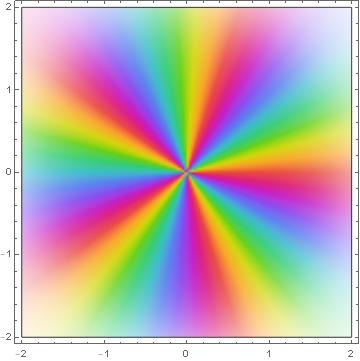# Complex exponentials

Here’s something that comes up occasionally, a case where I have to tell someone “It doesn’t work that way.” I’ll write it up here so next time I can just send them a link instead of retyping my explanation.

## Rules for exponents

The rules for manipulating expressions with real numbers carry over to complex numbers so often that it can be surprising when a rule doesn’t carry over. For example, the rule

(bx)y = bxy

holds when b is a positive real number and x and y are real numbers, but doesn’t necessarily hold when x or y are complex. In particular, if x is complex,

(ex)y = exy

does not hold in general, though it does hold when y is an integer. If it did hold, and this is where people get into trouble, you could make computations like

ei/3 = (ei)1/3 = 11/3 = 1

even though ei/3 actually equals (-1 + i√3)/2.

## Complex exponential function

I usually use the notation exp(x) rather than efor several reasons. For one thing, it’s easier to read. When the argument is complicated, say if it contains exponents of its own, the superscripts can get tiny and hard to head.  For example, here are two ways of writing the probability density function for the Gumbel distribution.Math publishers often require the exp() notation in their style guides.

Aside from legibility, there is a substantive reason to prefer the exp() notation. The function f(z) = exp(z) has a rigorous definition in terms of a power series. It’s a single-valued function, valid for any complex number you might stick in. By contrast, it’s subtle how bx can even be defined rigorously, even if be. If you care to delve into the details, see the footnote .  This may seem pedantic, but it’s a hair worth splitting because it can avoid difficulties like the incorrect calculation above.

Now it is true that

exp(xy) = exp(x) exp(y)

for all x and y, even complex x and y, and so it is true that

exp(nx) = exp(x)n

when n is an integer. For positive integers you can see this by repeatedly adding x to itself. You can extend this to negative integers using

exp(-x) = 1/exp(x).

## Related posts

 For positive b, you can bootstrap your way by defining bx for integer exponents, then rational exponents, then real exponents by taking limits. But when b is negative this doesn’t work. The way you end up defining bx in general is as exp(x log b), taking advantage of the rigorous definition of exp() alluded to above.

But what is log b? This gets subtle. The logarithm of b is a solution to the equation exp(x) = b, and this has infinitely many solutions for complex x. So now you need to either specify a particular branch to make the log function single valued, or you need to change your idea of a function to include multiply-valued functions.

If you get really technical, there’s a difference between exp(x) and ex is because the latter is exp(x log e), and log e has multiple values! So in that sense exp(x) and ex are different functions, the former single valued and the latter multiply valued.

# Gamma function partial sums

Last week I wrote about Jentzsch’s theorem. It says that if the power series of function has a finite radius of convergence, the set of zeros of the partial sums of the series will cluster around and fill in the boundary of convergence.

This post will look at the power series for the gamma function centered at 1 and will use a different plotting style.

Here’s what the contours look like with 12 terms: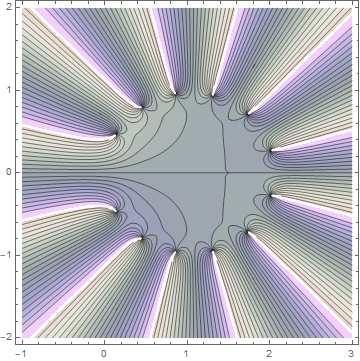The radius of convergence is 1 because the gamma function has a singularity at 0. (The radius of convergence is the distance from the center of the power series to the nearest singularity.) Contour lines correspond to constant phase. The gamma function is real for real arguments, and so you can see the real axis running through the middle of the plot because real numbers have zero phase. The contour lines meet at zeros, which you can see are near a circle of radius 1 centered at z = 1.

Here’s what the contour plot looks like with 30 terms:And here’s what it looks like with just 5 terms: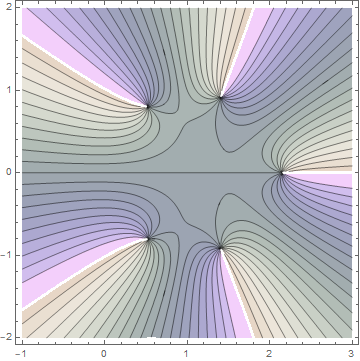Here’s the Mathematica code that was used to create the images.

    P[x_] = Normal[Series[1.0 Gamma[x], {x, 1, 12}]]
ContourPlot[
Arg[P[x + I y]],
{x, -1, 3},
{y, -2, 2},
ColorFunction -> "PearlColors",
Contours -> 20
]


The 1.0 in front of the call to Gamma is important. It tells Mathematica to numerically evaluate the coefficients of the power series. Otherwise Mathematica will find the coefficients symbolically and the plots will take forever.

Similarly, the call to Normal tells Mathematica not to carry around a symbolic error term O(x - 1)13.